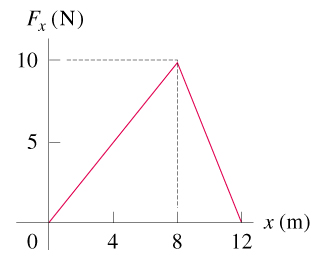# Problem: A child applies a force F parallel to the x-axis to a 8.00-kg sled moving on the frozen surface of a small pond. As the child controls the speed of the sled, the x-component of the force she applies varies with the x-coordinate of the sled as shown in figure . Suppose the sled is initially at rest at x=0. You can ignore friction between the sled and the surface of the pond.You may want to review (Pages 183 - 189). For related problemsolving tips and strategies, you may want to view a Video Tutor Solution of Motion on a curved path.Use the work-energy theorem to find the speed of the sled at 5.0 m.Use the work-energy theorem to find the speed of the sled at 11.0 m.

⚠️Our tutors found the solution shown to be helpful for the problem you're searching for. We don't have the exact solution yet.

###### Problem Details
A child applies a force F parallel to the x-axis to a 8.00-kg sled moving on the frozen surface of a small pond. As the child controls the speed of the sled, the x-component of the force she applies varies with the x-coordinate of the sled as shown in figure. Suppose the sled is initially at rest at x=0. You can ignore friction between the sled and the surface of the pond.

You may want to review (Pages 183 - 189).

For related problemsolving tips and strategies, you may want to view a Video Tutor Solution of Motion on a curved path.

Use the work-energy theorem to find the speed of the sled at 5.0 m.

Use the work-energy theorem to find the speed of the sled at 11.0 m.